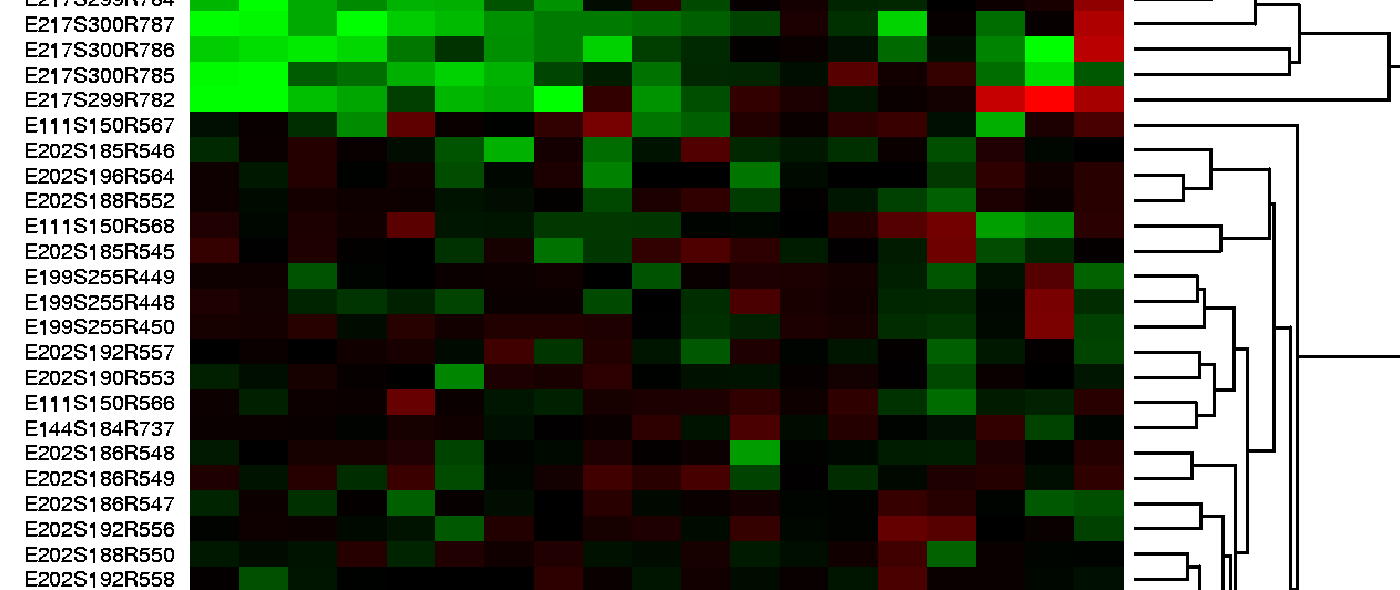Data Science Institute

# Homomorphic Encryption

Research Project

An encryption scheme is a method for efficiently computing an encrypted form e(X) of a given input X. It should be invertible, but computing the inverse must require a secret key.For the scheme to be secure, it should be hard to learn anything useful about a series of inputs given only the corresponding series of outputs. For over 30 years it was an open problem to find a secure encryption function that is homomorphic, which means that it satisfies the following extra properties: e(X + Y) = e(X) + e(Y) and e(XY) = e(X)e(Y). Among the many potential applications for such an encryption scheme would be the ability to perform calculations in the cloud on vast quantities of health data that has been encrypted for privacy. This project is focused on finding efficient approaches to homomorphic encryption.